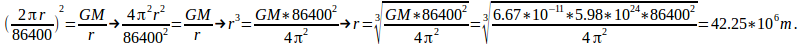A body in geostationary orbit orbits the Earth once every twenty four hours – the same time that the Earth takes to spin once – so stays above the same point on the Earth's equator.

We can find the radius of geostationary orbit by first equating the centripetal force equal to the gravitional force.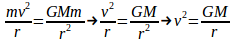(1)

where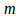is the mass of the satellite,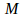is the mass of the Earth,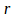is the radius of the orbit and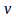is the speed of the satellite.

The satellite orbits once every 24 hours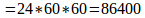seconds and travels a distance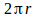so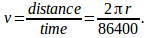Substitute this into (1) to obtain